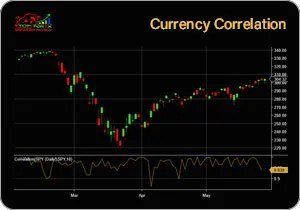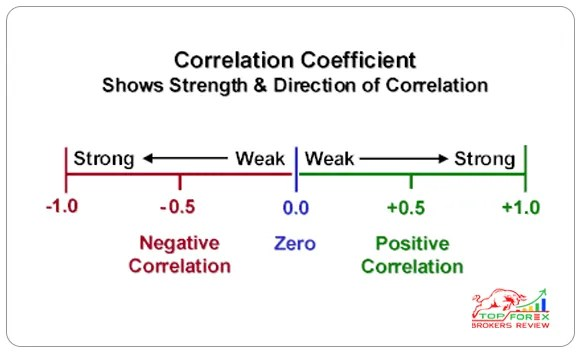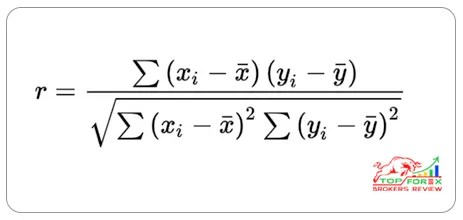TOP FOREX BROKERS REVIEW

THE INTERNET'S MOST COMPREHENSIVE LIST OF ONLINE FOREX BROKERS AND FOREX REVIEWS.

# Currency Correlations Explained, Factoring in Currency, Calculate Currency Correlations

What Is Forex Currency Correlations?The currency correlation is the statistical measure of how two currencies move in relation to each other. Thus, this relation tells us in which direction the two currency pairs will move in the given period of time.

Currencies are traded in pairs. There is a base currency and a quote currency. Unless you are planning to trade just one pair, it is mandatory that you learn and understand how currency pairs move in relation to one another. Currency correlations can greatly impact the amount of risk you are exposing your trading account to.

Correlation is computed into what is known as the correlation coefficient, which ranges between -1 and +1.

What Is Correlation Coefficient in Forex?

In forex trading, the correlation coefficient is a statistical measure that quantifies the relationship between two currency pairs. It indicates the degree to which the price movements of two currency pairs are synchronized or move in the same or opposite directions over a given period of time.

Correlation is calculated into a correlation coefficient that ranges between -1 and +1. A perfect positive correlation indicates that two currency pairs will move in the same direction all the time. A perfect negative correlation indicates that two currency pairs will move in the opposite direction all the time. Zero correlation means that the movement between the two currency pairs will have no correlation and they will have independent movement.

How to Read Currency Correlation Tables?

Reading currency pairs correlation tables is not that difficult. Don’t believe us? Have a look at the following table. The table the relationship between the main currency pair and other currency pairs in different time zones or frames.Table: EUR/USD Correlations

What you need to memorize is that currency pair correlation is represented in decimal format by a correlation coefficient, simply a number between -1.00 and +1.00. We have discussed the positive and negative signs on these numbers in our previous lessons.

+1 coefficient suggests that the two pairs will have a strong positive correlation and they are highly likely to move in the same direction. Similarly, -1 coefficient suggests that the two pairs still have a strong negative correlation and they are more likely to move in the opposite directions. A coefficient near or at zero indicates a very weak or random relationship. This type of relationship means that the currencies will not move in the same or opposite directions but random movements will be seen.

Using a forex correlation calculator will help you measure those correlations effectively.Correlation Coefficient Formulawhere:

r represents the correlation coefficient.

X and Y are the values of the two currency pairs being analyzed.

X̄ and Ȳ are the mean values of X and Y, respectively.

Σ denotes the sum of the values.

To calculate the correlation coefficient, you would need to gather historical price data for both currency pairs over a specific time period. Then, you calculate the mean values (X̄ and Ȳ) of the price data. Next, you subtract the mean values from each individual data point and multiply them together. These values are then summed up. Additionally, you calculate the sums of the squared differences between each data point and the mean values for both currency pairs.

Finally, you divide the sum of the multiplied differences by the product of the square roots of the sums of squared differences. This will give you the correlation coefficient, which will be a value between -1 and +1, indicating the strength and direction of the relationship between the two currency pairs.

Different Types of Correlation Coefficient

There are several types of correlation coefficients commonly used to measure the relationship between variables. Here are the main types:

Linear Correlation Coefficient

The linear correlation coefficient in forex, also known as the Pearson correlation coefficient, is a statistical measure that calculates the strength of the relationship between the relative movements of two currency pairs. The coefficient ranges between -1 and +1.

• A correlation coefficient of +1 indicates a perfect positive correlation. This means that the two currency pairs will move in the same direction 100% of the time. For example, if the EUR/USD and GBP/USD pairs have a correlation coefficient of +1, if EUR/USD goes up, GBP/USD will also go up.
• A correlation coefficient of -1 indicates a perfect negative correlation. This means that the two currency pairs will move in opposite directions 100% of the time. For example, if the EUR/USD and USD/CHF pairs have a correlation coefficient of -1, if EUR/USD goes up, USD/CHF will go down.
• A correlation coefficient of 0 means there is no correlation between the pairs – their movements are completely independent and random from each other.

Traders use this information to manage their portfolio and reduce risk. For instance, if two currency pairs have a high positive correlation and a trader has a long position in one, they may choose not to take a long position in the other to avoid doubling their risk. Similarly, knowing that two pairs are negatively correlated can help traders hedge their bets.

Sample Correlation Coefficient

In forex, the sample correlation coefficient refers to a statistical measure that quantifies the relationship between two currency pairs based on a sample of data. It is used to estimate the correlation between the two currency pairs in the broader forex market.

The sample correlation coefficient is denoted by “r” and is calculated using the same formula as the Pearson correlation coefficient. This coefficient ranges from -1 to +1, where +1 indicates a perfect positive correlation, -1 indicates a perfect negative correlation, and 0 indicates no correlation.

Spearman’s Rank Correlation Coefficient

The Spearman’ Rank Correlation Coefficient in forex trading is a way to figure out how two things are connected without using complicated math. It helps us see if there’s a strong or weak relationship between two currency pairs. Instead of looking at the exact numbers, it focuses on the order or ranking of the data points. For example, if one currency pair goes up and the other goes up too, or if one goes down and the other goes down, we say there’s a positive correlation. If one goes up while the other goes down, we call it a negative correlation. The Spearman Correlation Coefficient helps us understand these relationships in a simpler way.

The Spearman Correlation Coefficient is especially useful in forex trading because it can handle both linear and non-linear relationships. This makes it more versatile than the Pearson correlation coefficient, which can only handle linear relationships.

Matthews Correlation Coefficient

The Matthews Correlation Coefficient (MCC) is a measure of the quality of binary (two-class) classifications. It takes into account true and false positives and negatives and is generally regarded as a balanced measure which can be used even if the classes are of very different sizes.

In the context of forex trading, the MCC could be used to evaluate the performance of a trading strategy that makes binary decisions – for example, a strategy that decides whether to buy or sell a currency pair based on certain signals or conditions.

The MCC is computed as:

MCC = (TPTN – FPFN) / sqrt((TP+FP)(TP+FN)(TN+FP)*(TN+FN))

where:

TP = True Positives

TN = True Negatives

FP = False Positives

FN = False Negatives

The MCC returns a value between -1 and +1. A coefficient of +1 represents a perfect prediction, 0 an average random prediction and -1 an inverse prediction.

However, it’s important to note that the MCC is not commonly used in forex trading.

Currency correlation, as discussed earlier, help us know whether the two currency pairs will move in the same, opposite, or completely random direction over a given period of time. It is always important to remember that currencies are traded in pairs and no single currency pair is totally isolated.

Correlation is computed into a correlation coefficient that ranges between -1 and +1. Let’s give you a guide to interpreting the different correlation coefficient values.

Value    Interpretation

-0.1        Perfect Inverse Correlation

-0.8        Very Strong Inverse Correlation

-0.6        Strong Inverse Correlation

-0.4        Moderate Inverse Correlation

-0.2        Weal Inverse Correlation

0              Random or Zero Correlation

0.2          Weak Insignificant Correlation

0.4          Weak Low Correlation

0.6          Moderate Correlation

0.8          Strong High Correlation

1.0          Perfect Correlation

Now you are well prepared to read the currency correlation through a fancy chart. We know how much you are interested in knowing how currency correlations can make your trading more successful. Here are a few reasons why you need this skill in your arsenal.

Using correlations help you avoid positions that cancel out each other. Operating a position long in relation to a long other pair is not only pointless but expensive at times as well. Moreover, paying for the spread for the second time will make one movement in the price up for one pair and down for the other one.

Leverage Profits and Losses

You have the opportunity to maximize on positions to make maximum profits. Here, let’s have a look at the 1-week EUR/USD and GBP/USD relationship from the example discussed in the previous lesson.

These two pairs have a strong positive correlation with GBP/USD just behind EUR/USD. Opening a long position for each pair is simply like taking EUR/USD and increasing your position in the market for the given period of time.

In this case, you are simply making use of leverage! If things go right, you will be making more profit and vice versa.

Varying Risk

Understanding that relationships exist likewise enables you to utilize diverse money sets, yet at the same time influence your perspective. As opposed to trading a single currency pair constantly, what you can do is spread your risk over the two currency sets that move in a similar direction.

Or, you can also choose pairs that have a strong correlation (around 0.7). Let’s suppose, EUR/USD and GBP/USD will in general move together. The weak connection between these two currency pairs provides you the chance to expand which diminishes your risk. Suppose you’re bullish on USD.

Rather than opening two short places of EUR/USD, you could short one EUR/USD and short on GBP/USD which would shield you from some risk and broaden your general position. If the U.S. dollar sells off, the euro may be influenced to a lesser degree than the pound.

Despite the fact that supporting can bring about acknowledging littler benefits, it can offset the misfortunes. On the off chance that you open a long EUR/USD position and it begins to conflict with you, open a little long position in a couple that moves in the opposite direction EUR/USD, for example, USD/CHF.

You can exploit the distinctive pip esteems for every currency pair. For instance, while EUR/USD and USD/CHF have a practically flawless – 1.0 inverse relationship, their pip esteems are extraordinary. If you have any confusions regarding the movement of the currency pairs,

Accepting you trade a 10,000 small scale parcel, one pip for EUR/USD rises to \$1 and one pip for USD/CHF rises to \$0.93. In the event that you get one scaled-down part EUR/USD, you can HEDGE your trade by getting one smaller than expected parcel of USD/CHF. On the off chance that EUR/USD falls 10 pips, you would be down \$10. In any case, your USD/CHF trade would be up to \$9.30.

Rather than being down \$10, presently you’re just down \$0.70! Despite the fact that supporting sounds like the best thing since cut bread, it has a few hindrances. In the event that EUR/USD energizes, your benefit is constrained on account of the misfortunes from your USD/CHF position. Additionally, the relationship can weaken whenever. Envision if EUR/USD falls 10 pips, and USD/CHF just goes up 5 pips, remains level, or falls too!

Confirm Breakouts

Currency correlations can be used to confirm your trade entry or exit signals.

For example, the standard EUR/USD appears to be testing a crucial support level. You are looking at the price action and looking to sell on a breakout.

As you know that EUR/USD is in strong correlation with GBP/USD and negatively correlated with USD/CF and USD/JPY. What you need to see is that if the other pairs are moving in the same direction as EUR/USD as well.

What you should know that prices may actually trade under the support level and you have been observing it as well simply because the other three pairs are trading in proportion with EUR/USD.

If you are still keen to trade this setup, you should play it safe and trade with a smaller position to keep the risk minimum.

How to Calculate Currency Correlations with Excel?

Calculating currency correlations in Excel involves the same process as calculating any other type of correlation. Here’s a step-by-step guide:

1. Collect your data: You’ll need historical exchange rate data for the two currencies you want to analyze. You can usually download this data from financial websites. Make sure the data is in the same time frame (daily, weekly, monthly, etc.).
2. Input your data into Excel: Put the data for each currency pair in separate columns. For example, you could put the EUR/USD data in column A and the GBP/USD data in column B.
3. Calculate the correlation: Use the CORREL function in Excel to calculate the correlation. The syntax for the CORREL function is =CORREL(array1, array2). In this case, array1 and array2 are the ranges of cells containing the data for the two currency pairs. For example, if you have data in cells A2 through A100 and B2 through B100, you would use the formula =CORREL(A2:A100, B2:B100).
4. Interpret the result: The CORREL function will return a value between -1 and 1. A value of 1 means the two currency pairs move in the same direction most of the time (positive correlation), a value of -1 means they move in opposite directions most of the time (negative correlation), and a value of 0 means there is no correlation.

Remember, correlation does not imply causation. Just because two currency pairs have a high correlation does not mean changes in one cause changes in the other. Also, correlations can change over time, so it’s a good idea to update your analysis periodically.

Scroll To Top